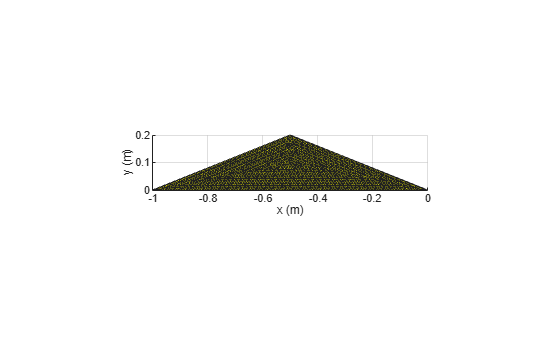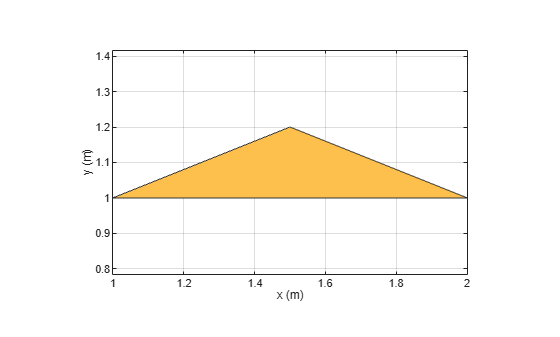# translate

Move shape to new location

## Syntax

``c = translate(shape,locationpoints)``

## Description

example

````c = translate(shape,locationpoints)` moves the shape to a new specified location using a translation vector.```

## Examples

collapse all

Create a polygon using `antenna.Polygon` with vertices at `[-1 0 0;-0.5 0.2 0;0 0 0]` and view it.

`p = antenna.Polygon('Vertices', [-1 0 0;-0.5 0.2 0;0 0 0])`
```p = Polygon with properties: Name: 'mypolygon' Vertices: [3x3 double] ```
```show(p) axis equal```Mesh the polygon and view it.

`mesh(p,0.2)`Move the polygon to a new location on the X-Y plane.

```translate(p,[2,1,0]) axis equal```## Input Arguments

collapse all

Shape created using custom elements and shape objects of Antenna Toolbox, specified as an object.

Example: `c = translate(rectangle1,[2 1 0])` where rectangle1 is created using `antenna.Rectangle` object.

Translation vector, specified as a vector.

Data Types: `double`

## Version History

Introduced in R2017a